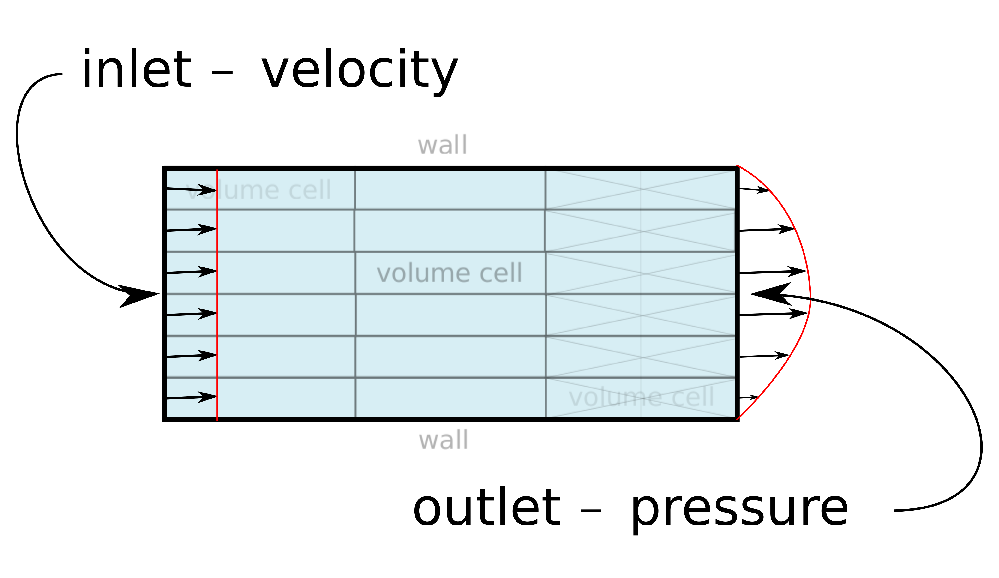This is an automatically generated documentation by LaTeX2HTML utility. In case of any issue, please, contact us at info@cfdsupport.com.

## Boundary conditions ​

• Boundary conditions are set in solution directories (0/U, …)

• The most typical case is the velocity is prescribed at the inlet and static pressure is prescribed at the outlet.Figure: Test case geometry

• Boundary conditions in OpenFOAM are divided into two groups by – basic and derived.
• Basic boundary conditions by OpenFOAM implementation:

 Boundary condition Description Variables fixedValue constant value at boundary value fixedGradient constant gradient gradient zeroGradient zero gradient (extrapolation) – calculated depending on other quantities – mixed mix from other boundary conditions refValue, refGradient, valueFraction, value directionMixed anisotropic BC mixed, refValue, refGradient, valueFraction is tensor valueFraction, value

• OpenFOAM has many derived boundary conditions
• Some examples follow
• Derived boundary conditions are stored on the path:
• \$FOAM_SRC/finiteVolume/fields/fvPatchFields/derived
• or can be found by following command:
# find \$FOAM_SRC -name "*derivedFvPatch*"
# find \$FOAM_SOLVERS -name "*derivedFvPatch*"

• Derived boundary conditions based on fixedValue

 name Description Variables totalPressure total pressure is constant,static pressure is computed pressureInletVelocity if known pressure at inlet value velocity is computed from flux pressureDirectedInletVelocity if known pressure at inlet value, velocity is computed from flux inletDirection velocity direction is respected movingWallVelocity moving wall value surfaceNormalFixedValue velocity magnitude is kept, value direction is perpendicular to domain turbulentInlet calculates a fluctuating referenceField variable based on a scale of fluctuationScale a mean value

• Derived boundary conditions based on fixedGradient/zeroGradient boundary condition

 Name Description Variables fluxCorrectedVelocity Normal component is computed value wallBuoyantPressure Sets pressure gradient value value according to atmospheric pressure

• Derived boundary conditions based on mixed boundary condition

 Name Description Variables inletOutlet switching between fixedValue inletValue, and zeroGradient value depending on flow direction outletInlet switching between fixedValue outletValue, and zeroGradient value depending on flow direction pressureInletOutletVelocity combination of pressureInletVelocity value and inletOutlet pressureDirectedInletOutletVelocity combination of value, pressureDirectedInletVelocity and inletOutlet pressureTransmissive transmitting supersonic pressure waves pInf supersonicFreeStream transmits shocks to surroundings pInf, TInf, UInf

• Another derived boundary conditions

 Name Description Variables slip slipping wall – partialSlip combines slip and zeroGradient valueFraction depending on valueFraction (0 = slip) noSlip fixes the velocity to zero – at the walls